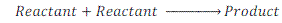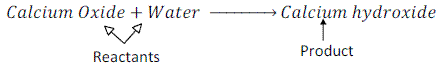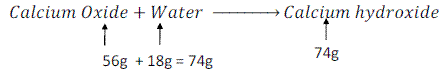Courses

# Atoms and Molecules - Introduction, Chapter Notes, Class 9, Science Class 9 Notes | EduRev

Created by: Himanshu Aryan

## Class 9 : Atoms and Molecules - Introduction, Chapter Notes, Class 9, Science Class 9 Notes | EduRev

The document Atoms and Molecules - Introduction, Chapter Notes, Class 9, Science Class 9 Notes | EduRev is a part of Class 9 category.
All you need of Class 9 at this link: Class 9

## Laws of Chemical Combinations

Before Dalton concept of atom was mere philosophical. Dalton explained about atom on the basis of Laws of Chemical Combinations.

There are three laws of chemical combination.

1. Law of Conservation of Mass
2. Law of Constant Proportions
3. Law of Multiple Proportions

### Law of Conservation of Mass

Antoine L. Lavoisier, a French scientist, established the theory of Law of Conservation of Mass.

The law of conservation of mass states, “Mass can neither be created nor destroyed in a chemical reaction”.

According to this law mass of an isolated system will remain constant over time. This means when mass is enclosed in a system and none is allowed in or out, its quantity will never change. That is mass will be conserved, and hence this is called Law of Conservation of Mass. This means total mass of products is always equal to the total mass of reactants. As there is no loss of mass of substances, i.e. mass is conserved, that’s why Lavoisier called this the law of conservation of mass.

Reactants and Products:

In a chemical reaction the substances that combine or react are known as reactants and the new substance/substances formed are called product or products.

A chemical reaction can be represented in general as follows:Example:

When calcium oxide is dissolved in water calcium hydroxide is formed. The reaction invole in this can be written as:In this reaction calcium oxide and water are reactants while calcium hydroxide is product.

In this reaction 74 g of calcium hydroxide is obtained when 56 g of calcium oxide reacts with 18 g of water, which is proved by experiment.Here the total mass of reactants, i.e. calcium oxide and water is equal to 74 g. And the mass of product, i.e. calcium hydroxide is also equal to 74g. This proves that the total mass of reactants is always equal to the total mass of product, which proves the Law of Conservation of Mass.

#### Law of Constant Proportions

Law of Constant Proportion states that a chemical compound always contains exactly the same proportion of elements by mass.

This law is also known as Law of definite proportions. Joseph Louis Proust gave this law hence, this law is also known as Proust’s Law.

Explanation of the law:-

Compounds are formed by the combination of two or more elements. In a compound the ratio of the atoms or element by mass remains always same irrespective of the source of compound. This means a certain compound always formed by the combination of atoms in same ratio by mass. If the ratio of mass of constituent atoms will be altered the new compound is formed.

Examples:-

Water is formed by the combination of hydrogen and oxygen. The ratio of masses of hydrogen and oxygen is always in 1:8 in water irrespective of source of water. Whether you collect the water from a well, river, pond or from anywhere the ratio of their constituent atoms by mass will always same.

Nitrogen dioxide is a compound, which is formed by the combination of nitrogen and oxygen. The ratio of nitrogen and oxygen by mass in nitrogen dioxide is in 7:16.

Nitrous oxide is a compound which is also formed by the combination of nitrogen and oxygen. The ratio of nitrogen and oxygen in nitrous oxide is in 28:16.

Nitric oxide is a compound, which is also formed by the combination of nitrogen and oxygen. The ratio of nitrogen and oxygen in nitric oxide is in 7:8.

From the above three examples it is clear that if the ratio of the atoms by mass is altered then the new compound is formed, such as in the case of nitrogen dioxide, nitrous oxide, nitric oxide. These three compounds are formed by the combination of same atoms but because of combination of the constituent atoms in different ratios by mass new compound is formed.

##### Dalton’s Atomic Theory

John Dalton, a British Chemists and scientists gave the Atomic Theory in 1808. This theory is popularly known as Dalton’s Atomic Theory in the honour of John Dalton. He gave the theory on the basis of Laws of Chemical Combination and explains them properly. In his theory he explains about atom.

###### Main postulates of Dalton's atomic theory
1. Elements are made of extremely small particles called atoms.
2. Atoms of a given element are identical in size, mass, and other properties;
3. Atoms of different elements differ in size, mass, and other properties.
4. Atoms cannot be subdivided, created, or destroyed.
5. Atoms of different elements combine in simple whole-number ratios to form chemical compounds.
6. In chemical reactions, atoms are combined, separated, or rearranged.

,

,

,

,

,

,

,

,

,

,

,

,

,

,

,

,

,

,

,

,

,

,

,

,

,

,

,

,

,

,

;